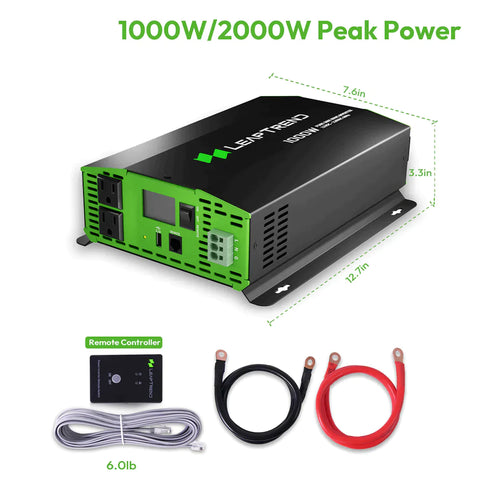# How Long Will a 12 Volt Battery Run a 1000 Watt Inverter?

If you're planning to use a 1000 watt inverter with a 12 volt battery, you may be wondering how long the battery will last. The answer depends on a few factors, including the capacity of the battery, the efficiency of the inverter, and the amount of power the device or devices you're running require.

Summary: If using a 12V 100Ah battery to power 1000W inverter at full load, the estimated runtime is approximately one hour.

## Battery Capacity

The first factor to consider is the capacity of your battery. Batteries are rated by their ampere hour (Ah) capacity, which is a measure of how much electrical charge the battery can store. For example, a 100 Ah battery can supply a current of 1 ampere for 100 hours, or 10 amperes for 10 hours.

So how does capacity relate to the question of how long a 12 volt battery will run a 1000 watt inverter? Using a little algebra, we can rearrange the formula P=IV to solve for time:

Time = capacity / (power / voltage)

In this case, the power is 1000 watts and the voltage is 12 volts. Let's assume you have a 100 Ah battery, which is a common size for a deep cycle battery. Plugging in the values, we get:

Time = 100 Ah / (1000 W / 12 V) = 1.2 hours

So with a 100 Ah battery and a 1000 watt inverter, you can expect to run your devices for about an hour and a half before the battery is drained.## Inverter Efficiency

Of course, this calculation assumes that the inverter is 100% efficient, which is unlikely. Inverters are not 100% efficient - some of the power is lost in the process of converting DC to AC. In general, you can expect an inverter to be about 80-90% efficient.

To factor in efficiency, we can multiply the calculated time by the inverter efficiency. For example, with an 80% efficient inverter, the runtime would be:

Time = 1.2 hours x 0.8 = 0.96 hours, or about 57 minutes.

## Power Requirements

Finally, the amount of power the device or devices you're running require will affect the runtime. If you're running a device that requires more power than the inverter can handle, the battery will be drained more quickly. Conversely, if you're running devices that require less power than the inverter can handle, you'll be able to run them for longer.

In general, it's a good idea to add up the power requirements of all the devices you plan to run and make sure the inverter you choose can handle the total load.So how long will a 12 volt battery run a 1000 watt inverter? The answer depends on the capacity of the battery, the efficiency of the inverter, and the power requirements of the devices you're running. A 100 Ah battery and an 80% efficient inverter should allow you to run your devices for about an hour, assuming they don't require more power than the inverter can handle. Remember to choose an inverter with enough capacity to handle your total load, and to factor in efficiency when calculating runtime.